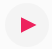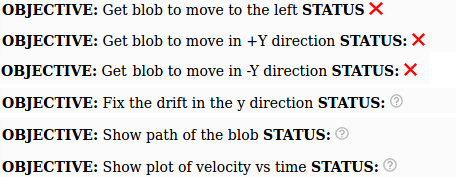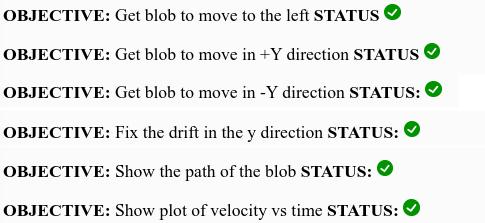# Your first video game: Move the blob!

On the internet there are some fun games where you control a circle-looking-thing that you can move around and interact (and potentially destroy or absorb!) other circle-looking things controlled by other people. Two games that come to mind are diep.io and agar.io. There are plenty of others like them out there.

Step 1. Look at a computer code for a blob that can only move in the x-direction!

In the top left corner, pressand you'll see something like this:Do what it says! Press the arrow keys on your keyboard to see if the blob moves! The blob will only move when you press right arrow! Your job will be to modify the computer program to make it move in other directions.

After you press all four arrow keys you will notice that there are a couple of objectives that have not yet been completed:If the program can detect that something is wrong with your code it will show a rednext to that objective.

If the program can detect that you have completed the objective it will show a green check marknext to that objective.

But the program is not very smart and if it can't tell whether you have completed the objective it will indicate a question mark like thisnext to that objective.

By the end of this activity your goal is to have green check marks next to all the objectives like this:Step 2. In the top right corner of the screen click "Sign up" (if you want to save your work)

If you want to save your work then create an account and confirm a password. Nobody will send you e-mails to bug you for doing this. Unfortunately you have to create an account in order to save your changes to the code. Please note that if you do not want to create an account the only way to save your code is to highlight your code with the mouse and copy paste it into a text file in MS Word or some other program.

Click Duplicate to copy a version of the code to your account

Step 3. Look at the code! Read the //comments in the code

Here's the code. Each section does something important. Bear in mind that draw() is run over and over again, 60 times per second in fact. This means that 60 times per second, the code will update the $x$ position, and check if the keyboard keys are being pressed.

function draw(){

// Update location
x += vx*dt;

// velocity is zero unless keys are pressed
vx = 0;

// Turn or thrust the ship depending on what key is pressed
if (keyIsDown(LEFT_ARROW)) {
// Do nothing
}
if (keyIsDown(RIGHT_ARROW)) {
vx = 10;
}
if (keyIsDown(UP_ARROW)) {
// Do nothing
}
if (keyIsDown(DOWN_ARROW)) {
// Do nothing
}

// Draw axes and other stuff
// This will clear the screen and re-draw it
display();

drawBlob(x,y,vx,vy);

// Add more graphics here before the end of draw()

} // end draw()   DO NOT ADD ANY CODE AFTER THIS LINE!!!


Your job will be to replace the "Do nothing" with something that will allow the blob to move in both the $x$ and the $y$ directions.

Step 4. Make the blob move to the left!

What happens when you change this section:

    if (keyIsDown(LEFT_ARROW)) {
// Do nothing
}


to this:

    if (keyIsDown(LEFT_ARROW)) {
vx = 10;
}


Now when you press the left arrow does it move the blob to the left? Why or why not? How might you fix this? (Hint: this seems to only move the blob in the positive x direction. If only there were some way to make it move in the negative x direction.)

Change the program until you can move the blob to the left or right as in this example

### Step 5. Modify the program to get the blob to move in the y direction

Step 5a. Modify the code so that vy = 10 if you press the up arrow

By now you've noticed that to get the blob to move in the +x direction when you press the right arrow, there is a section of the code that sets vx to 10 when you press the right arrow:

    if (keyIsDown(RIGHT_ARROW)) {
vx = 10;
}


Let's make sure something like this happens when you press the up arrow. Find this part of the code:

    if (keyIsDown(UP_ARROW)) {
// Do nothing
}


and replace // Do nothing with vy = 10;. Can this move the blob in the y direction when you press one of the arrow keys? (Hint: maybe not!)

Comment: Leave the down arrow section alone for now. We'll work on that later.

Step 5b. Make sure the x AND y position are getting updated!

In order for the blob to move in BOTH the x AND the y directions, we need to make sure that both the x and y positions are getting updated. This task has to do with this part of the code:

    // Update location
x += vx*dt;


Comment #1 x += vx*dt is how a computer thinks about this equation: $$d = vt$$

We like to think about the total distance ($d$) traveled after the total time ($t$) elapsed. Computers like to think about short periods of time that last dt seconds long, so that the total time is $t = N \cdot dt$ where $N$ is the number of short periods of time and $dt$ is the number of seconds (or fraction of a second) of one of these short periods of time.

Comment #2 dt is short for "delta t" = $\Delta t$. In other words the "d" in dt comes from the greek symbol for "delta". dt is not a distance, it is a short period of time.

Let's look at the section in the code again:

    // Update location
x += vx*dt;


You'll notice that there is nothing about y here. The only thing that gets updated is the x position. This is why in the previous step the y position didn't change even though the we set vy = 10 in Step 4a.

Add a line to the code above make sure the y position gets updated if vy is non-zero (Hint: it will look a lot like how we update the x position of the blob.

If you do this successfully your code should behave like this

Step 6. Fix the drift!

If you play around with the code at the end of Step 5 you will probably notice that once the blob starts moving in the y direction, there's nothing you can do to stop this. In other words, even when you're not pressing the up arrow, the blob will drift upwards.

Look closely at the code and see if you can figure out what's causing this. (Hint: there's a section between the "update location" and the keyboard section that we haven't modified yet). You should only need to add one line of code to fix the drift!

If you can fix the drift, your code should behave like this

Step 7. Let the blob move in the negative y direction!

If you haven't already, modify the code so that pressing the down arrow moves the blob in the negative y direction. You would need to modify this part of the code:

    if (keyIsDown(DOWN_ARROW)) {
// Do nothing
}


Hint: This is basically the same problem we ran into in Step 4. If only we could make the blob move in the negative y direction instead of the positive y direction.

If you make the right change your code should behave like this

Step 8. Add code to show the path of the blob

After display(); you can add this code and it will show the path of the blob:

    for( i = 0; i < xhistory.length ; i+= 1) {
drawPoint(xhistory[i],yhistory[i]);
}


Press the button above to copy the code to the clipboard. In the code editor press Control+V to paste it into the code.

When the blob changes direction is the path smooth and curvy or does the path have sharp corners? How realistic is this for real life?

Step 9. Add a graph of $v_x$ versus time!

You can add a plot of $v_x$ versus time by adding this code to the program. You can put it anywhere after the display() function

graph1.addPoint(vx);
graph1.display();


Press the button above to copy the code to the clipboard. In the code editor press Control+V to paste it into the code.

Does the plot make sense to you? What does it look like a bunch of flat lines or rectangles?

### At this point your code should have green check marks for all the objectives! Yay!

Note: Just for fun you can add a plot of $v_y$ versus time

graph2.addPoint(vy);
graph2.display();


Step 10. Give the blob an initial velocity!

Try this out: At the beginning of the program change this code:

x = 375;
y = 250;

vx = 0;
vy = 0;

to this:
x = 0;
y = 0;

vx = 25;
vy = 25;


In the draw function comment out or delete these lines:

// velocity is zero unless keys are pressed
vx = 0;
vy = 0;


Now what happens when you run the program? Does it make sense?

### Challenge Question:

1. Why does it seem like the arrows are longer when the blob is moving in a diagonal direction? Is the blob really moving at a faster speed?

### Next Steps

Accelerate the blob:

See if you can modify this Accelerate the blob example so that instead of only moving in the x direction, the blob can accelerate in both the x and the y directions

Dan Shiffman's Agar.io clone:

If you're feeling ambitious you can work through Dan Shiffman's step-by-step tutorial to re-create agar.io